Homefrom Alom Shaha on Vimeo.

"The most incomprehensible thing about the universe is that
it is comprehensible."

- Einstein

Over Arching Concept

If a mass speeds up, slows down or changes direction,
a net Force Must Be Present - Newton

Video Instruction

showmethephysics.com

 Questions to Investigate 1. How is speed different than velocity? 2. How is speed measured? 3. How long is a meter? 4. About how fast are do you walk? (m/s) 3. How is displacement different than distance?

Unit I - Mechanics - Study of the relationship between force and motionI. Kinematics - Mathematical methods of describing and predicting motion

Describe motion by using:

A) Average Speed

Average Speed
of 120 miles per hour

can also be written ...

120 miles/hr

per = divided by

Could mean travelled

120 miles in one hour

OR ....

60 miles in ...

1/2 hour (30 minutes)

30 miles in ...

15 minutes

OR 15 miles

in 7.5 minutesCan you believe the speed limit in
!!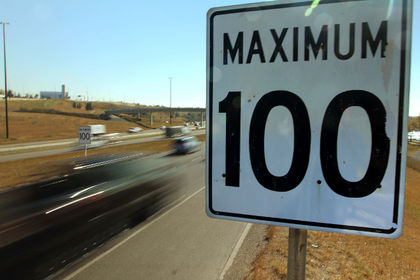100 km/hr  ≈ 60 mph

Avg. Speed - how much distance an object covers per unit time

1)
Units: m/s, km/hour,

miles/hour

Average Speed = Δd/Δt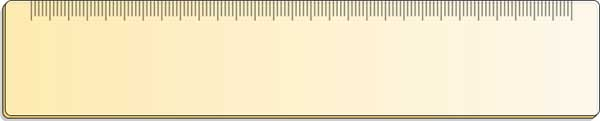Distance

(meter ≈ 1 yard)

(km ≈ .6 miles)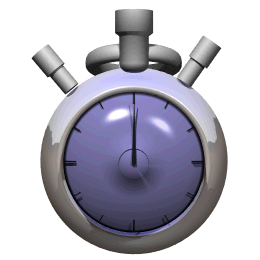Time
- secs, minutes, hours

 Ex 1) An object travels 5.0 meters in 2.0 seconds.   Find its speed.

Speed = Δd/Δt

= 5.0 m/2.0 sec

= 2.5 m/s

 Ex 2) An object moves at a rate of 3.0 m/s for 1.5 seconds.   How far did the object move?

Speed =Δd/Δt

3.0 m/s = Δd/1.5 sec

Δd = 4.5 m

Motion Map

3.0 m/s for 1.5 seconds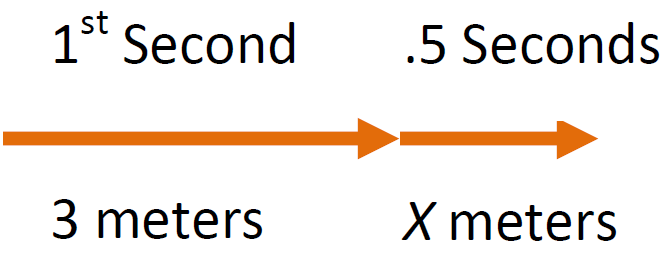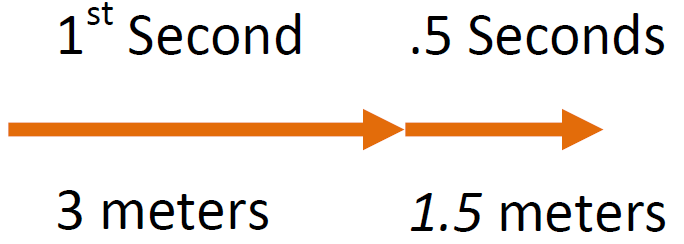Δd = 4.5 m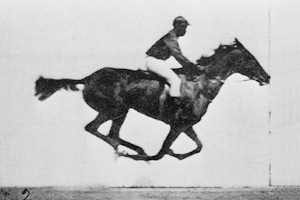Animation by User Waugsberg

Public Domain

 Ex 3) An object moves at rate of 4.0 m/s over a distance of 10. meters.   How long did it take to travel that distance? t = ?

Speed = d/t

4.0 m/s = 10. m/t

(Cross Multiply)

(4.0 m/s)t = 10. m

(Divide by 4)

t = 2.5 seconds

Motion Map

How much time to travel 10 m @ 4 m/s?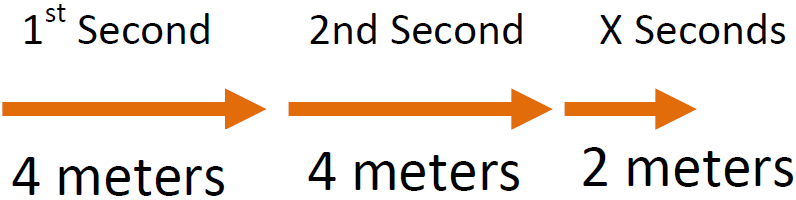t = 2.5 secondsMotion Plots I